# Maharashtra Board 12th Commerce Maths Solutions Chapter 5 Index Numbers Ex 5.3

Balbharati Maharashtra State Board 12th Commerce Maths Solution Book Pdf Chapter 5 Index Numbers Ex 5.3 Questions and Answers.

## Maharashtra State Board 12th Commerce Maths Solutions Chapter 5 Index Numbers Ex 5.3

Calculate the cost of living index in problems 1 to 3.

Question 1.Solution: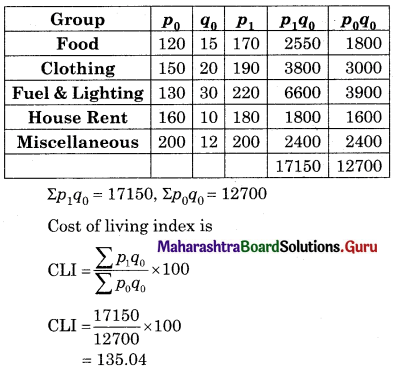Question 2.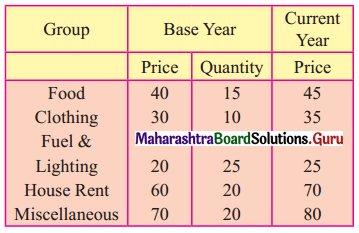Solution: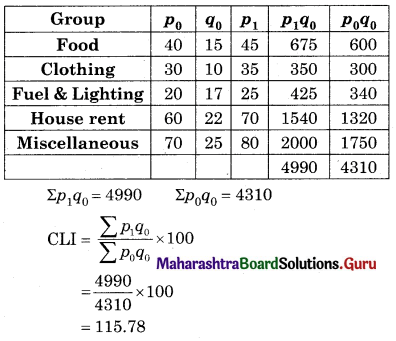Question 3.Solution:Base year weights (W) and current year price relatives (I) are given in problems 4 to 8. Calculate the cost of living index in each case.

Question 4.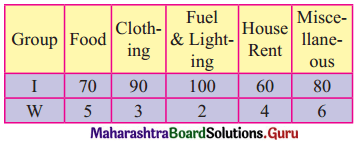Solution:ΣW = 20, ΣIW = 1540
CLI = $$\frac{\sum \mathrm{IW}}{\sum \mathrm{W}}$$
= $$\frac{1540}{20}$$
= 77

Question 5.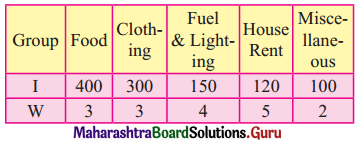Solution: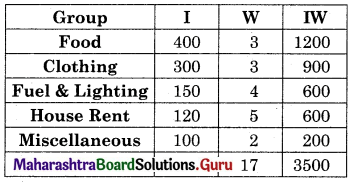ΣW = 17, ΣIW = 3500
CLI = $$\frac{\sum \mathrm{IW}}{\sum \mathrm{W}}$$
= $$\frac{3500}{17}$$
= 205.88Question 6.Solution: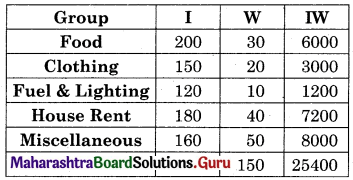ΣW = 150, ΣIW = 25400
CLI = $$\frac{\sum \mathrm{IW}}{\sum \mathrm{W}}$$
= $$\frac{25400}{150}$$
= 169.33

Question 7.Solution:ΣW = x + 18, ΣIW = 100x + 3600
CLI = 150
∴ $$\frac{\sum \mathrm{IW}}{\sum \mathrm{W}}$$ = 150
∴ $$\frac{100 x+3600}{x+18}$$ = 150
∴ 100x + 3600 = 150x + 2700
∴ 50x = 900
∴ x = 18

Question 8.
Find y if the cost of living index is 200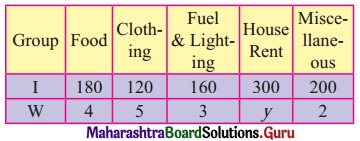Solution:ΣW = y + 18, ΣIW = 300y + 2200
CLI = 200
∴ $$\frac{\sum \mathrm{IW}}{\sum \mathrm{W}}$$ = 200
∴ $$\frac{300 y+2200}{y+14}$$ = 200
∴ 300y + 2200 = 200y + 2800
∴ 100y = 600
∴ y = 6Question 9.
The cost of living Index numbers for years 1995 and 1999 are 140 and 200 respectively. A person earns ₹ 11,200 per month in the year 1995. What should be his monthly earning in the year 1999 in order to maintain his standard of living as in the year 1995?
Solution:
CLI (1995) = 140
CLI (1999) = 200
Income (1995) = 11200
Income (1999) = ?
For year 1995
∴ Real Income = $$\frac{\text { Income }}{\text { CLI }} \times 100$$
= $$\frac{11200}{140}$$ × 100
= ₹ 8000
For year 1999
∴ Real Income = $$\frac{\text { Income }}{\text { CLI }} \times 100$$
∴ 8000 = $$\frac{\text { Income } \times 100}{200}$$
∴ Income = 16000
∴ Income in 1999 = ₹ 16000